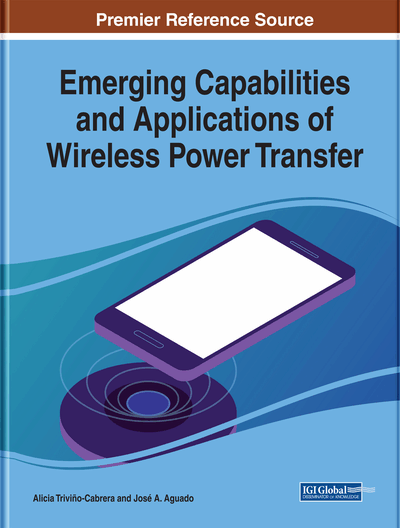# Resonant Compensations in Inductive Wireless Power System

Alberto Reatti (University of Florence, Italy), Marian K. Kazimierczuk (Wright State University, USA), Agasthya Ayachit (Wright State University, USA) and Fabio Corti (University of Florence, Italy)
DOI: 10.4018/978-1-5225-5870-5.ch003

## Abstract

A resonant wireless power system uses capacitors to create resonance between primary and secondary coil at a specific resonant frequency. Thereby, they reduce the reactive power and produce the required transmitted real power over a long range of separation and at higher efficiencies. Depending on the connection between the capacitors and coils, four main topologies can be identified: series-series, series-parallel, parallel-series, and parallel-parallel. In this chapter, these resonance compensations schemes are analyzed, and the advantages and disadvantages of each topology are highlighted.
Chapter Preview
Top

## Introduction

One of the most important feature of an Inductive Wireless Power System (IWPS) is the power transmission efficiency. An IWPS consists of two coupled inductors called primary and secondary coils. If the primary coil is supplied by an alternating current and/or voltage, a time-varying magnetic fieldis produced. A part of this magnetic field, according to the coupling coefficient between the primary and secondary coils reaches the secondary coil and, therefore, an electro motive force is induced cross the secondary coil turns. A higher part of the magnetic field produced on the primary reaches the secondary, the higher is the voltage across the secondary coil terminals and, for any given load resistance, the higher is the output power delivered to the load. Unfortunately, non-resonant coupled inductors waste most of energy in the form of conduction losses in the primary coil, also for a very short range and a large coupling (Spanik, FrivaldskyDragona, & Jaros, 2016). The efficiency is actually drastically improved and a wider transmission distance is achieved if the primary and secondary are tuned by utilizing the resonance principle (Chopra, Bauer, 2011; Sirbu, Mandache; & Kazimierczuk, 2014). When an IWPS is considered, the resonance is achieved by utilizing capacitors. This allows Resonant Wireless Power System (RWPS) to be designed and built. These capacitors are designed to create resonance between the primary and secondary coils at a specific resonant frequency; thereby, they reduce the reactive power circulating inside the circuits and can allow the transmission of real power over relatively large distances among the primary and secondary coils with increased efficiencies (Zhang, 2015). More exactly, the main tasks of compensating circuits are as follows:

• 1.

To introduce a secondary side reactance able to resonate with the secondary side reactance of the native circuit. Since the Inductive Wireless Power Systems are based on the magnetic coupling of two coils (which results in equivalent inductances), the resonating reactance is constituted by a capacitor.

• 2.

The reactance introduced on the primary side adds to the native circuit input impedance and must result in a pure resistive equivalent circuit seen by the input voltage source. In Inductive Wireless Power Systems, the secondary side circuit added to the primary side inductance results to be an R-L circuit. Therefore, a capacitor is also needed on the primary side to cancel the reactive part of the input impedance.

As a result, the compensating networks studied in this chapter are constituted by one primary side capacitor C1 utilized on the circuit primary side and a capacitor C2 on the circuit secondary side (Mohamed & Berzoy, 2017).

Depending on the connection between the capacitors and coils, four main topologies can be identified: Series - Series, Series - Parallel, Parallel - Series, and Parallel - Parallel. In the following section, these resonance compensations schemes are analyzed, and the advantages and disadvantages of each topology are highlighted.

Top

## Assumptions

The analysis of all the compensations topologies is carried out under the following assumptions:

## Complete Chapter List

Search this Book:
Reset